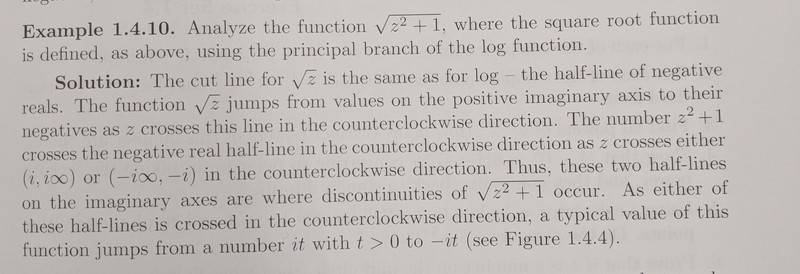# Complex Analysis - sqrt(z^2 + 1) function behavior

## Homework Statement## Homework Equations

The relevant equation is that sqrt(z) = e^(1/2 log z) and the principal branch is from (-pi, pi]

## The Attempt at a Solution

The solution is provided, since this isn't a homework problem (I was told to post it here anyway). I don't understand why the number z^2 + 1 crosses the negative real half-line in the counterclockwise direction as z crosses (i, i inf). Is it because z^2 + 1 where z = i is 0? I would think the argument of z^2 + 1 should be around pi in order to cross the negative real half line, but I'm not sure. Also, I'm not sure how they said the value jumps from it to -it when the half lines are crossed.